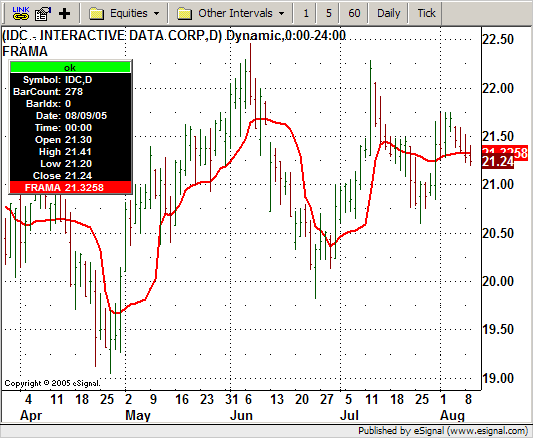# 2005 Oct: Fractal Adaptive Moving Averages (FRAMA.efs)

ICE Data Services -

FRAMA.efs

File Name: FRAMA.efs

Description:
This formula is based on the October 2005 article, Fractal Adaptive Moving Averages, by John F. Ehlers.

Formula Parameters:

• Length: 16

Notes:
The study also has one parameter for the Length, or periods, for the study that may be adjusted through the Edit Studies option of the Advanced Chart. The number entered will be forced to be the next highest even number if an odd number is entered. The related article is copyrighted material. If you are not a subscriber of Stocks & Commodities, please visit www.traders.com.

FRAMA.efsEFS Code:

```/***************************************
Provided By : eSignal (c) Copyright 2005
Description:  Fractal Adapative Moving Average - by John Ehlers

Version 1.0  8/9/2005

Notes:
October 2005 Issue - "FRAMA - Fractal Adaptive Moving Average"

* Study requires version 7.9 or higher.
* Length will be forced to be an even number.  Odd numbers will be
bumped up to the next even number.

Formula Parameters:                 Defaults:
Length                              16
***************************************/

function preMain() {
setPriceStudy(true);
setStudyTitle("FRAMA ");
setShowTitleParameters(false);
setCursorLabelName("FRAMA", 0);
setDefaultBarFgColor(Color.red, 0);
setDefaultBarThickness(2, 0);

var fp1 = new FunctionParameter("nLength", FunctionParameter.NUMBER);
fp1.setName("Length");
fp1.setDefault(16);
fp1.setLowerLimit(1);
}

var bVersion = null;

var Filt = null;
var Filt_1 = null;   //previous bar's Filt

function main(nLength) {

if (bVersion == null) bVersion = verify();
if (bVersion == false) return;

var nState = getBarState();

if (nState == BARSTATE_NEWBAR) {
Filt_1 = Filt;
}

var N = Math.round(nLength/2) * 2; // forces N to be even number
var Price = hl2();
var count = 0;
var N1 = 0;
var N2 = 0;
var N3 = (highest(N, high()) - lowest(N, low())) / N;
var HH = high(0);
var LL = low(0);
var Dimen = 0;
var alpha = 0;
Filt = 0;

if (Filt_1 == null) Filt_1 = 0;

for( count = 0; count <= (N/2 -1); count++) {
if (high(-count) > HH) HH = high(-count);
if (low(-count) < LL) LL = low(-count);
}

N1 = (HH - LL) / (N / 2);
HH = high(-(N/2));
LL = low(-(N/2));

for (count = (N/2); count <= (N-1); count++) {
if (high(-count) > HH) HH = high(-count);
if (low(-count) < LL) LL = low(-count);
}

N2 = (HH - LL) / (N / 2);

if (N1 > 0 && N2 > 0 && N3 > 0) {
Dimen = (Math.log(N1 + N2) - Math.log(N3)) / Math.log(2);
}

alpha = Math.exp(-4.6*(Dimen - 1));

if (alpha < 0.01) alpha = 0.01;
if (alpha > 1) alpha = 1;

Filt = (alpha*Price) + (1 - alpha)*Filt_1;

if (getCurrentBarCount() < N) Filt = Price;

return Filt;
}

/***** Support Functions *****/

function verify() {
var b = false;
if (getBuildNumber() < 700) {
drawTextAbsolute(5, 35, "This study requires version 7.9 or later.",
Color.white, Color.blue, Text.RELATIVETOBOTTOM|Text.RELATIVETOLEFT|Text.BOLD|Text.LEFT,
null, 13, "error");